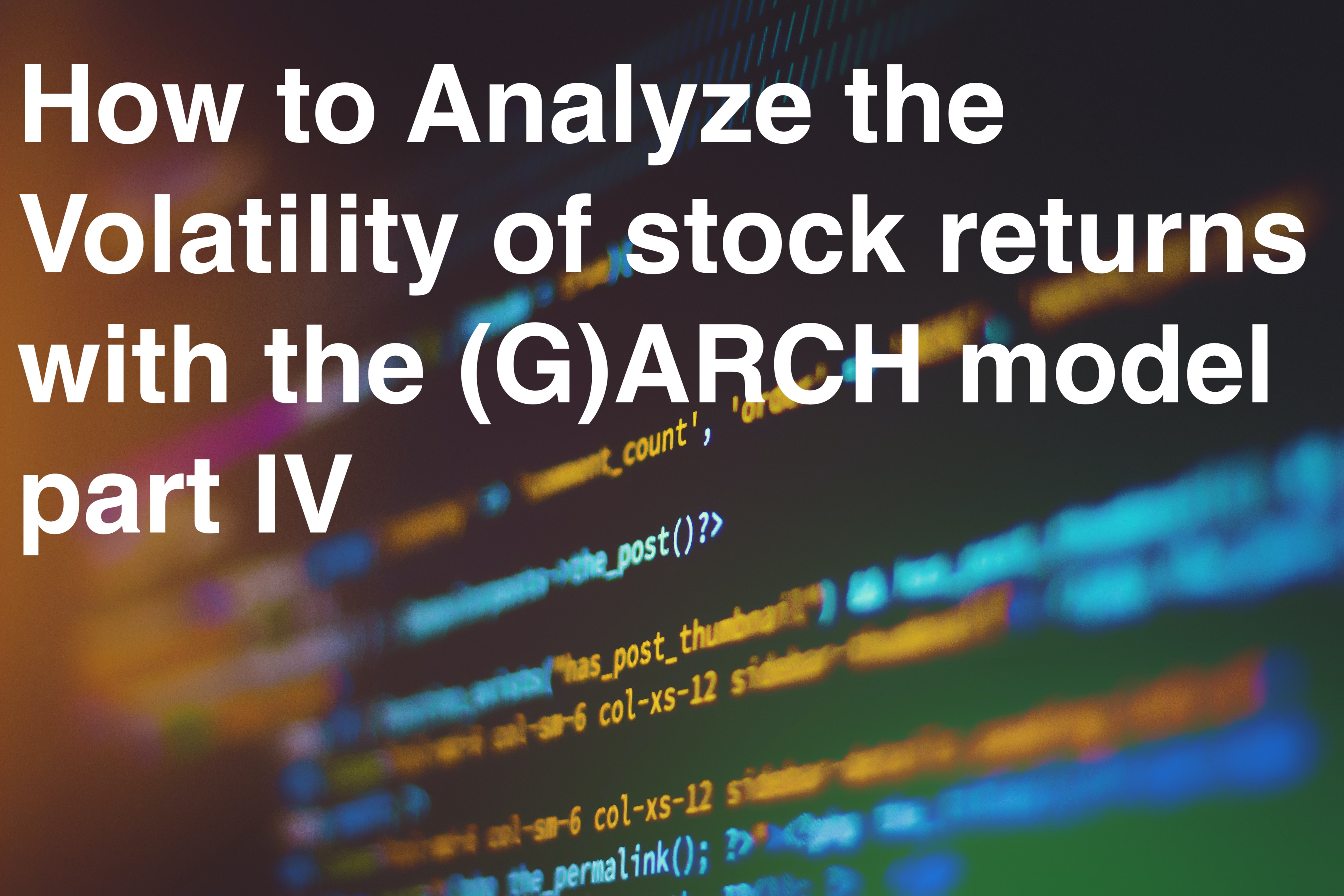10
min

# How to Analyze the Volatility of stock returns with the (G)ARCH model part IV

Analysis of the AR (Auto-Regressive) Model.### 4.3 AR (Auto-Regressive) Model

The result of the PACF can now be used in the calculation of the AR models for Nasdaq100and gold. For the Nasdaq100, it is advisable to calculate an AR(9) model, since here you have the first 9 lags in the model and after the PACF all of them, except lag 3 and 5, are significant.With gold, there is a choice between lag 17 and lag 29. Since lag 17 already contains many estimators, you should choose lag 17 here, since it can also be assumed that no significant result can be expected from many estimators. So an AR(17) model should be modeled for gold. Figure 1.8 shows the result of the AR(9) model for the Nasdaq100 log return. The result reflects the expected result that the lags that already indicated significance in the PACF now also form significant estimators in the AR model.

The estimators ar1, ar2, ar6, ar7, ar8 and ar9 are significant because they have a high t-value.The estimators ar3 and ar5 are not significant and ar4 is in the limit. The constant is also significant. So the estimators ar1, ar2, ar6, ar7, ar8 and ar9 have a significant impact on Y(t). Figure 1.9 shows the AR(17) model for gold's log return. As expected, many estimators do not show significant results. The only significant estimator is ar17, as expected from thePACF. Ar17 (Y_(Yt-17 )) is the only significant influence on Y(t).

Looking at the residuals for skewness and kurtosis, left skewness and increased kurtosis can still be seen.

### 4.4 Comparison of the residuals of the AR models

Figure 2.1 shows the comparison of the residuals from the AR(9) model for the Nasdaq100 and the AR(17) model for gold. Figure 2.2 shows the squared residuals of both models.Looking at the results of the log return analysis, one sees similar results. Gold fluctuates less again and forms different extremes than the Nasdaq100. This effect can be seen in both graphs. Since the structure is still similar to that of returns, it can still be assumed that there is no normal distribution and that the time series is still left-skewed and that there is a leptokurtic distribution (Schmelzer 2009: 30).

if you want to continue reading go to part V

#### Sources:

Marcus Schmelzer, (2009), The volatility of financial market data, theoretical foundations and empirical analyzes of hourly return time series and risk measures, Universität Köln

Image: from Shahadat Rahman at Unsplash## Joshua Denefleh

CQO & Co-Founder

Strong background in financial markets, graduate from University Mannheim in B.Sc. Economics, KIT Karlsruhe University. Experience in quantitative methods, programming algo /w python